﻿ Coincidence Points and Common Fixed Points for Four Self-Mappings via Weakly Compatible Mappings in Cone Metric SpacesPublications are Open
Access in this journal
Article Versions
Export Article
• Normal Style
• MLA Style
• APA Style
• Chicago Style
Research Article
Open Access Peer-reviewed

### Coincidence Points and Common Fixed Points for Four Self-Mappings via Weakly Compatible Mappings in Cone Metric Spaces

K. Prudhvi
American Journal of Applied Mathematics and Statistics. 2017, 5(3), 112-114. DOI: 10.12691/ajams-5-3-5
Published online: September 07, 2017

### Abstract

In this paper, we obtain coincidence points and common fixed point theorem for four self-mappings via weakly compatible mappings in cone metric spaces, where the cone is not necessarily normal. These results are improved and generalized several well- known comparable results existing in the references.

### 1. Introduction

Huang and Zhang 8 introduced the concept of a cone metric space, they replaced set of real numbers by an ordered Banach space and proved some fixed point theorems for single mapping for contractive type conditions in cone metric spaces, using the normality condition. Later on many authors have 1, 2, 3, 4, 6, 7, 9, 10, 11 generalized and extended the results of Huang and Zhang 8 and obtained the common fixed points for two or more self-mappings for different types of contractive conditions in cone metric spaces with and without using the normality condition. In this paper, we obtained coincidence points and common fixed points for four self-mappings in cone metric spaces without the normality condition. Our results are extended and improved the results of Arshad, Azam and Vetro 5.

The following definitions are due to Huang and Zhang 8.

Definition 1.1. 8

Let B be a real Banach space and P be a subset of B. The set P is called a cone if and only if:

(a). P is closed, non–empty and P ≠ {0};

(b). a,b ∈ℝ, a, b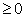, x,y∈P ⇒ ax+by∈P;

(c). P ∩ (-P) = {0}.

Definition 1.2. 8

Let P be a cone in a Banach space B, define partial ordering ‘≤’ with respect to P by x ≤ y if and only if y-x∈P. We shall write x<y to indicate x ≤ y but x ≠ y while x<<y will stand for y-x∈ int P, where, int P denotes the interior of the set P. This cone P is called an order cone.

Definition 1.3. 8 Let B be a Banach space and P ⊂ B be an order cone. The order cone P is called normal if there exists M > 0 such that for all x, y ∈ B,The least positive number M satisfying the above inequality is called the normal constant of P.

Definition 1.4. 8 Let X be a nonempty set of B Suppose that the map d: X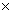X→ B satisfies:

(d1). 0 < d(x, y) for all x, y ∈ X with x ≠ y and d(x, y) = 0 if and only if x = y;

(d2). d(x, y) = d(y, x) for all x, y ∈ X;

(d3). d(x, y)≤ d(x, z) + d(z, y) for all x, y, z ∈ X.

Then d is called a cone metric on X and (X, d) is called a cone metric space.

It is clear that the concept of a cone metric space is more general than that of a metric space.

Example 1.5. 8 Let B = ℝ2, P = {(x, y)∈B such that: x, y ≥ 0} ⊆ ℝ2, X = ℝ and d: X × X → B such that d(x , y) = (│x - y│, α│x - y│), where α ≥ 0 is a constant. Then (X, d) is a cone metric space.

Definition 1.6 8 Let (X, d) be a cone metric space .We say that {xn} is said to be

(i) a convergent sequence if for any c>>0, there is a natural number N such that for all n>N, d(xn, x) <<c, for some fixed x in X. We denote this xn→x (as n→∞).

(ii) a Cauchy sequence if for every c in E with c>>0, there is a natural number N such that for all n, m>N, d(xn, xm) << c.

(iii) a cone metric space (X, d) is said to be complete if every Cauchy sequence in X is convergent.

Definition 1.7. 5 A pair (S, T) of self-mappings on X is said to be weakly compatible if they commute at their coincidence point ( i. e., STx = TSx), whenever Sx = Tx).

### 2. Main Results

In this section, we prove a result on points of coincidence and common fixed points for self-mappings and then show that this result generalizes some of the recent results existing in the literature.

Lemma 2.1. Let X be a nonempty set and the mappings S, T, I and J: X→ X point of coincidence p in X. If {S, I} and {T, J} are weakly compatible if they commute at their coincidence p in X. If {S, I} and {T, J} are weakly compatibles, then S, T, I and J have a unique common fixed point.

Proof. Since, p is a coincidence point of S, T, I and J. Therefore p = Iq = Jq = Sq = Tq for some q∈ X. By weakly compatibility of {S, I} and {T, J} we have Sp = SIq = ISq = Ip, Tp = TJq = JTq = Jp.

It implies that Sp = Tp = Ip = Jp = r(say), then r is a point of coincidence of S,T, I and J. Therefore, p = r by uniqueness. Thus p is a unique common fixed point of S, T, I and J.

We adopt the technique which was used in 5.

Let (X, d) be a cone metric space and S, T, I and J be self-mappings on X such that S(X) ⊆ J(X), T(X) ⊆ I(X). Suppose x0 ∈ X and x1, x2 ∈ X is chosen such that S(x0) = J(x1), T(x1) = I(x2) continuing this process we can define {xn} by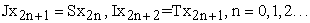Denote,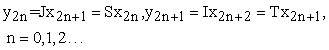(1)

The sequence {yn} is called an S-T-sequence with initial point x0.

The following result is an extension of Proposition 3.2,. in 5.

Proposition 2.2. Let (X, d) be a cone metric space and P be an order cone. Let S, T, I, and J:X→X be such that S(X) ⊆ J(X), T(X) ⊆ I(X). Assume that the following conditions hold:

(i) d(Sx, Ty) ≤ α d(Ix, Sx) + β d(Jy, Ty) + γ d(Ix, Jy), for all x, y∈X with x ≠ y, where α, β, γ are non-negative real numbers with α + β + γ < 1;

(ii) d(Sx, Tx) < d(Ix, Sx) + d(Jx, Tx) , for all x∈X, whenever Sx ≠ Tx.

Then every S-T-sequence with initial point x0∈X is a Cauchy sequence.

Proof. Let x0 be an arbitrary point in X and {yn} be an S-T-sequence with initial point x0 . First we assume that y2n ≠ y2n+1 for all n. Then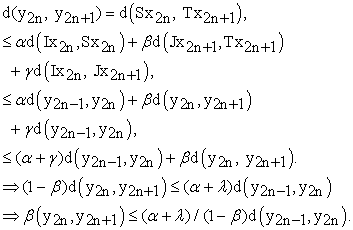(2)

Similarly, we obtain that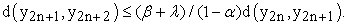(3)

Now from (2) and (3), we deduce that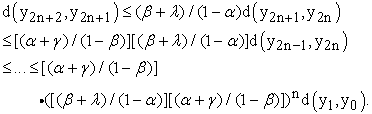(4)

And(5)

Let M = (α + γ) /(1-β), N = (β + γ) /(1-α).

Then MN < 1. Now for any n > m, we have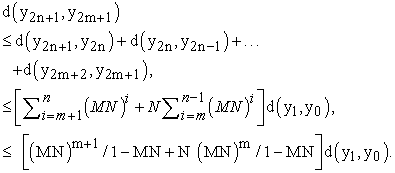In analogous way, we againAnd d(y2n+1, y2m) ≤ (N+1) (MN)m+1 / 1-MN d(y1, y0).

Thus, for n > m > 0,For each c >> 0, choose δ > 0 such that c – x∈int P, where ||x||<< δ, that is, x << c, for this δ, we can choose a natural number N1 such that ||bm d(y1, y0)||< δ, for m > N1 .

Thus we get that d(yn, ym) ≤ bm d(y1, y0) << c, for all n > m > N1. Therefore {yn} is a Cauchy sequence in (X, d). Now we suppose that y2n = y2n+1 for some n∈N1. If x2n = x2n+1 , by (ii) we have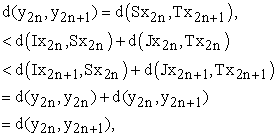which implies that y2n = y2n+1.

If x2n ≠ x2n+1 , we use (i) to obtain y2n = y2n+1.

Similarly, we deduce that y2n+1 = y2n+2 and so yn = ym for every n ≥ m. Hence, {yn} is a Cauchy sequence.

Theorem 2. 3. Let (X, d) be a cone metric space and P be an order cone, let S, T, I and J: X → X be such that S(X) ⊆ J(X), T(X) ⊆ I(X). Assume that the following conditions are hold.

(i) (Sx, Ty) ≤ α d(Ix, Sx) + β d(Jy, Ty) + γ d(Ix, Jy), for all x, y∈X with x ≠ y, where α, β, γ are non-negative real numbers with α + β + γ < 1;

(ii) d(Sx, Tx) < d(Ix, Sx) + d(Jx, Tx) , for all x∈X, whenever Sx ≠ Tx.

If one of S(X), T(X), I(X) and J(X) is a complete subspace of X, then S, T and f have a unique point of coincidence. Moreover, if {S, I} and {T, J} are weakly compatible, then S, T, I and J have a unique common fixed point.

Proof. Let x0 be an arbitrary point in X. By Proposition 2.2 every S-T-sequence {yn} with initial point x0 is a Cauchy sequence. Suppose J(X) is complete there exists p∈ J(X) such that y2n = Sx2n = Jx2n+1 → p as n→∞. We can find a u∈X such that Ju = p (If S(X) is complete, there exists p∈S(X)⊆J(X) , then the conclusions remain the same). Now we show that Tu = p. By (i), we have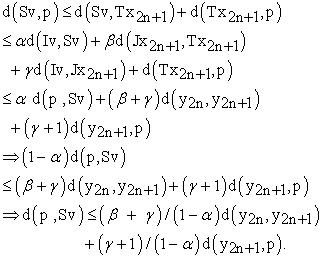Fix 0<<c and choose n1 ∈N be such that d(y2n-1, y2n) << (1- α)/(β + γ) c/2, and d(y2n, p) << (1- α)/( γ+1) c/2 for all n ≥ n1.

Consequently, d(p, Sv) << c/2 + c/2 = c.

⇒ d(p, Sv) << c and hence d(p, Sv) << c/m for every m∈N. From c/m – d(p, Sv) ∈int P, being P is closed as m→∞, we deduce –d(p, Sv)∈P and so d(v, Sp) = 0.

⇒ p = Sv.

Therefore, Sv = p = Iv.

Therefore, Tu = Ju = Sv = Iv (= p).

⇒ p is a point of coincidence of S, T, I and J have a unique point of coincidence. For his we assume that there exists another point p* in X such that p* = Tu* = Tu* = Sv* = Iv*, then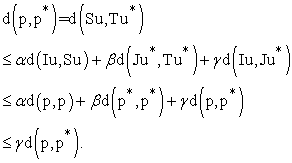We deduce p = p*. Since, {S, I} and {T, J} are weakly compatible, then p is the unique point of coincidence of S, T, I and J then by the above Proposition we get that p is the unique common fixed point of S, T, I and J.

Remark 2.4.

(i) If we choose I = J = f in the above Theorem 2.3., we can get the Theorem 3.3., of 5.

The above Theorem 2.3., generalizes the Theorem 3.3., of 5.

(ii) If we choose S = T and I = J = f in the above Theorem 2.3., we can get the Theorem 3.4., of 5.

The above Theorem 2.3., generalizes the Theorem 3.4., of 5.

### References

  M. Aamri and D. E. Moutawakil, Some new common fixed point theorems under strict contractive conditions, J. Math. Anal. Appl. 270, 181-188, 2002. In article View Article  M. Abbas and G. Jungck, Common fixed point results for non commuting mappings without continuity in cone metric spaces, J. Math. Anal. Appl. 341, 416-420, 2008. In article View Article  M. Abbas and B. E. Rhoades, Fixed and periodic point results in cone metric spaces, Appl. Math. Lett. Vol.22, no.4, 511-515, 2009. In article View Article  I. Altun, and G. Durmaz, Some fixed point theorems on ordered cone metric spaces, Rend. Ciric. Mat. Palermo 58, 319-325, 2009. In article View Article  M. Arshad, A. Azam and P. Vetro, Some common fixed point results in cone metric spaces, Fixed Point Theory and Applications, Volume, Article ID493965, 11, 2009. In article View Article  P. N. Dutta and B. S. Choudary, A generalization of contraction principle in metric spaces, Fixed Point Theory Appl. 2008, Article ID 406386, 8, 2008. In article View Article  O. Ege and I. Karaca, Nielsen fixed point theory for digital images, Journal of Computational Analysis and Applications, 22(5), 874-880, 2017. In article View Article  L. G. Huang, X. Zhang, Cone metric spaces and fixed point theorems of contractive mappings, J. Math. Anal. Appl. 332(2), 1468-1476, 2007. In article View Article  D. IIic, V. Rakocevic, Common fixed points for maps on cone metric space, J. Math. Anal. Appl. 341, 876-882, 2008. In article View Article  Sh. Rezapour and R. Hamlbarani, Some notes on the paper cone metric spaces and fixed point theorem of contractive mappings, J. Math. Anal. Appl. 345, 719-724, 2008. In article View Article  P. Vetro, Common fixed points in cone metric spaces, Rendiconti del Circolo Matematico di Palermo, 56(3), 464-468, 2007. In article View ArticleThis work is licensed under a Creative Commons Attribution 4.0 International License. To view a copy of this license, visit http://creativecommons.org/licenses/by/4.0/